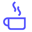a concise shot of dev knowledge
Become a Contributor
Concise shots of dev knowledge

RELATED TAGS

graph
data structures

# What is a graph (data structure)?Edpresso Team

A graph is a common data structure that consists of a finite set of nodes (or vertices) and a set of edges connecting them.

A pair (x,y) is referred to as an edge, which communicates that the x vertex connects to the y vertex.

In the examples below, circles represent vertices, while lines represent edges.

Graphs are used to solve real-life problems that involve representation of the problem space as a network. Examples of networks include telephone networks, circuit networks, social networks (like LinkedIn, Facebook etc.).

For example, a single user in Facebook can be represented as a node (vertex) while their connection with others can be represented as an edge between nodes.

Each node can be a structure that contains information like user’s id, name, gender, etc.Graph showing a Social Network (Nodes as users and Edges show connection)

## Types of graphs:

### Undirected Graph:

In an undirected graph, nodes are connected by edges that are all bidirectional. For example if an edge connects node 1 and 2, we can traverse from node 1 to node 2, and from node 2 to 1.

### Directed Graph

In a directed graph, nodes are connected by directed edges – they only go in one direction. For example, if an edge connects node 1 and 2, but the arrow head points towards 2, we can only traverse from node 1 to node 2 – not in the opposite direction.